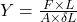## Ten identical steel wires have equal lengths L and equal “spring constants” k. The Young’s modulus of each wire is Y. The wires are connecte

Question

Ten identical steel wires have equal lengths L and equal “spring constants” k. The Young’s modulus of each wire is Y. The wires are connected end to end, so that the resultant wire has length 10L. What is the Young’s modulus of the resulting wireA) 0.1YB) YC) 10YD) 100Y

in progress 0
6 months 2021-07-29T20:23:52+00:00 1 Answers 8 views 0

option (B)

Explanation:

Young’s modulus is defined as the ratio of longitudinal stress to the longitudinal strain.

Its unit is N/m².

The formula for the Young’s modulus is given bywhere, F is the force applied on a rod, L is the initial length of the rod, ΔL is the change in length of the rod as the force is applied, A is the area of crossection of the rod.

It is the property of material of solid. So, when the 10 wires are co joined together to form a new wire of length 10 L, the material remains same so the young’ modulus remains same.